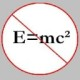You are currently browsing the tag archive for the ‘prime’ tag.

I found this elementary number theory problem in the “Problem Drive” section of Invariant Magazine (Issue 16, 2005), published by the Student Mathematical Society of the University of Oxford. Below, I have included the solution, which is very elementary.

Problem: Find all ordered pairs of prime numbers$(p,q)$ such that$p^q + q^p$ is also a prime.

Solution: Let$E = p^q+q^p$. First, note that if$(p,q)$ is a solution, then so is$(q,p)$. Now,$p$ and$q$ can’t be both even or both odd, else$E$ will be even. Without loss of generality, assume$p = 2$ and$q$ some odd prime. So,$E = 2^q + q^2$. There are two cases to consider.

Case 1:$q = 3$.

This yields$E = 2^3 + 3^2 = 17$, which is prime. So,$(2,3)$ and, hence$(3,2)$ are solutions.

Case 2:$q > 3$.

There are two sub-cases to consider.$1^{\circ}:$$q = 3k+1$, where$k$ is some even integer. Then, we have$E = 2^{3k+1} + (3k+1)^2 \equiv (-1)^k(-1) + 1 \equiv -1 + 1 \equiv 0 \pmod 3$. Hence,$3 \mid E$; so,$E$ can’t be prime.$2^{\circ}:$$q = 3k+2$, where$k$ is some odd integer. Then we have$E = 2^{3k+2} + (3k+2)^2 \equiv (-1)^k(1) + 1 \equiv -1 + 1 \equiv 0 \pmod 3$. Hence,$3 \mid E$; so, again,$E$ can’t be prime.

As we have exhausted all possible cases, we conclude$(2,3)$ and$(3,2)$ are the only possible solutions.

• 355,659 hits

### Recent CommentsJohn Favors on Solution to POW-13: Highly…Wayne J. Mann on Solution to POW-12: A graph co…erneststephen on The 54th Carnival of Math…anhtraisg on p^q + q^p is primeprof dr drd horia or… on My first postprof drd horia orasa… on My first postprof dr mircea orasa… on Inequality with lognotedscholar on Self-referential Paradoxes, In…prof dr mircea orasa… on Inequality with logprof dr mircea orasa… on Inequality with logprof dr mircea orasa… on 2010 in reviewkenji on Basic category theory, Iprof dr mircea orasa… on Solution to POW-10: Another ha…prof drd horia orasa… on Continued fraction for eprof drd horia orasa… on Inequality with log